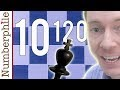To anyone who has ever complained that a game of chess is boring, we can at least guarantee you this: Every game you play will be different. How do we know? Well, it's estimated that there are more possible iterations of a game of chess than there are atoms in the known universe. In fact, the number of possible moves is so vast that no one has ever been able to calculate it exactly.

In the 1950s, mathematician Claude Shannon wrote a paper about how one could program a computer to play chess. In it, he made a quick calculation to determine how many different games of chess were possible, and came up with the number 10^120. This is a very, very large number — the number of atoms in the observable universe, by comparison, is only estimated to be around 10^80.

Shannon's number came from a rough calculation that used averages instead of exact figures. It assumed that at any point in the game you'd have an average of 30 legal moves, for example, and that every game has an average of 80 total moves. But that's not how chess works. You have many fewer legal moves at the beginning of a game than the end, and games can go much shorter or longer than 80 moves.

There are other complications as well: even if you have 30 possible moves, only a few will make sense strategically. This is why it's such a challenge to calculate the number of possible games of chess, and why to this day, no one has landed on an exact figure. learn more about this in the video below:Share it:

Post A Comment